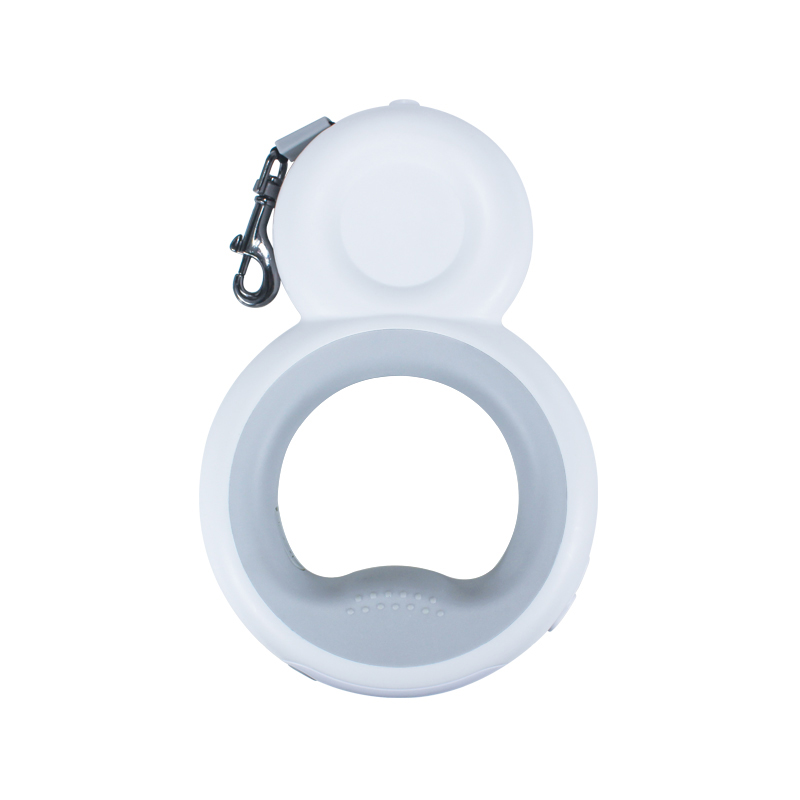• English
• United StatesпјҲUSD \$пјү
• \$ USD
• ВЈ GBP
• вӮ¬ EUR
• A\$ AUD
• вӮ№ INR
• ВҘ JPY
• ШҜ.ШҘ AED
• Af AFN
• L ALL
• Фҙ AMD
• Ж’ ANG
• Kz AOA
• \$ ARS
• Ж’ AWG
• РјР°РҪ AZN
• РҡРң BAM
• BBD BBD
• а§і BDT
• Р»РІ BGN
• вӮЈ BIF
• BD\$ BMD
• B\$ BND
• Bs. BOB
• BOV BOV
• R\$ BRL
• BSD BSD
• Nu. BTN
• P BWP
• Br BYN
• Be BZD
• вӮЈ CDF
• вӮЈ CHF
• CLP CLP
• ВҘ CNY
• \$ COP
• вӮЎ CRC
• \$ CUC
• \$ CUP
• \$ CVE
• KДҚ CZK
• вӮЈ DJF
• kr DKK
• \$ DOP
• ШҜ.Ш¬ DZD
• EВЈ EGP
• Nfk ERN
• Br ETB
• FJ\$ FJD
• FKP FKP
• бғҡ GEL
• вӮө GHS
• ВЈ GIP
• D GMD
• вӮЈ GNF
• Q GTQ
• \$ GYD
• HK\$ HKD
• L HNL
• Kn HRK
• G HTG
• Ft HUF
• Rp IDR
• вӮӘ ILS
• п·ј IRR
• Kr ISK
• \$ JMD
• Sh KES
• РЎМІ KGS
• бҹӣ KHR
• Fr KMF
• \$ KYD
• гҖ’ KZT
• вӮӯ LAK
• Щ„.Щ„ LBP
• Rs LKR
• L\$ LRD
• L LSL
• L MDL
• РҙРөРҪ MKD
• K MMK
• вӮ® MNT
• P MOP
• вӮЁ MUR
• Юғ. MVR
• MK MWK
• \$ MXN
• RM MYR
• MTn MZN
• вӮҰ NGN
• C\$ NIO
• kr NOK
• вӮЁ NPR
• \$ NZD
• B/. PAB
• S/ PEN
• K PGK
• вӮұ PHP
• вӮЁ PKR
• zЕӮ PLN
• вӮІ PYG
• Шұ.ЩӮ QAR
• L RON
• din RSD
• СҖ. RUB
• вӮЈ RWF
• Шұ.Ші SAR
• Si\$ SBD
• вӮЁ SCR
• Ш¬.Ші. SDG
• kr SEK
• S\$ SGD
• ВЈ SHP
• Le SLL
• Sh SOS
• \$ SRD
• SDP SSP
• Db STN
• Щ„.Ші SYP
• L SZL
• аёҝ THB
• Р…Рң TJS
• m TMT
• T\$ TOP
• вӮӨ TRY
• TTD TTD
• NT\$ TWD
• Sh TZS
• вӮҙ UAH
• Sh UGX
• \$ UYU
• Р»РІ UZS
• Bs.S. VES
• вӮ« VND
• Vt VUV
• T WST
• вӮЈ XAF
• \$ XCD
• Fr XOF
• вӮЈ XPF
• п·ј YER
• R ZAR
• ZK ZMW
• ШҜЩҠЩҶШ§Шұ KWD
• Шұ.Ш№. OMR
• .ШҜ.ШЁ BHD

No relevant currency found# TKC0101 Pet Leash with Bright LED Light

\$29.00 USD \$0.00 USD
Free worldwide shipping
Free returns
Carbon neutral
Secure payments
Color
Grey
Pink
Blue
Description